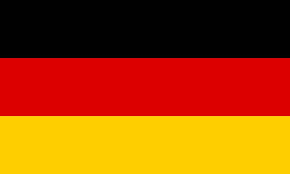# FunctionsCurve sketching
 Enter your function here. Hints: Enteras 3*x^2 ,as (x+1)/(x-2x^4) andas 3/5.

Linear functions
 Enter what you know about your linear function. Leave out the rest and Mathepower computes it. Equation: Slope: y-line intercept: Graph goes through points… Point A (|) Point B (|)

Find a function

Degree of the function:
 1 2 3 4 5

( The degree is the highest power of an x. )

Symmetries:
axis symmetric to the y-axis
point symmetric to the origin

y-axis intercept

Roots / Maxima / Minima /Inflection points:
at x=
at x=
at x=
at x=
at x=

Characteristic points:
at |)
at |)
at |)
at (|)
at (|)

Slope at given x-coordinates:
Slope at x=
Slope at x=
Slope at

 Transforming functions Enter your function here. How shall your function be transformed? By in x-direction stretched shrinked By in y-direction stretched shrinked By to the the right l the left By to the up down